# Weil algebra

Motivated by algebraic geometry, A. Weil [a3] suggested the treatment of infinitesimal objects as homomorphisms from algebras of smooth functionsinto some real finite-dimensional commutative algebrawith unit. The points in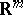correspond to the choice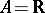, while the algebra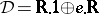,, of dual numbers (also called Study numbers) leads to the tangent vectors at points in(viewed as derivations on functions). At the same time, Ch. Ehresmann established similar objects, jets (cf. also Jet), in the realm of differential geometry, cf. [a1].

Since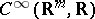is formally real (i.e.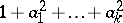is invertible for all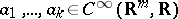), the values of the homomorphisms in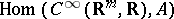are in formally real subalgebras. Now, for each finite-dimensional real commutative unital algebrawhich is formally real, there is a decomposition of the unit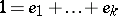into all minimal idempotent elements. Thus,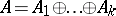, where, and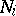are nilpotent ideals in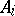. A real unital finite-dimensional commutative algebrais called a Weil algebra if it is of the form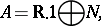whereis the ideal of all nilpotent elements in. The smallest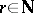with the property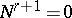is called the depth, or order, of.

In other words, one may also characterize the Weil algebras as the formally real and local (i.e. the ring structure is local, cf. also Local ring) finite-dimensional commutative real unital algebras. See [a2], 35.1, for details.

As a consequence of the Nakayama lemma, the Weil algebras can be also characterized as the local finite-dimensional quotients of the algebras of real polynomials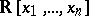. Consequently, the Weil algebrascorrespond to choices of idealsinof finite codimension. The algebra of Study numbers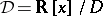is given by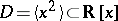, for example. Equivalently, one may consider the algebras of formal power series or the algebras of germs of smooth functions at the origin(cf. also Germ) instead of the polynomials.

The width of a Weil algebra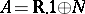is defined as the dimension of the vector space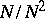. Ifis an ideal of finite codimension in,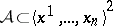, then the width of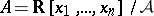equals. For example, the Weil algebra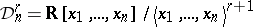has widthand order, and it coincides with the algebra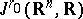of-jets of smooth functions at the origin in. Moreover, each Weil algebra of widthand orderis a quotient of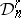.

Tensor products of Weil algebras are Weil algebras again. For instance,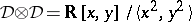.

The infinitesimal objects of typeattached to points inare simply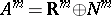. All smooth functions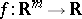extend to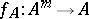by the evaluation of the Taylor series (cf. also Whitney extension theorem)where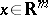,,are multi-indices,. Applying this formula to all components of a mapping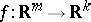, one obtains an assignment functorial in bothand. Of course, this definition extends to a functor on all locally defined smooth mappingsand so each Weil algebra gives rise to a Weil functor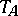. (See Weil bundle for more details.)

The automorphism group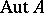of a Weil algebra is a Lie subgroup (cf. also Lie group) in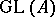and its Lie algebra coincides with the space of all derivations (cf. also Derivation in a ring) on,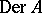, i.e. all mappingssatisfying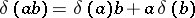, cf. [a2], 42.9.